Dr. S. R. Lasker Library Online Catalogue

Normal view

# John E. Freund's mathematical statistics with applications / Irwin Miller, Marylees Miller.

Material type:TextLanguage: English Publication details: c2014. Edition: 8th edDescription: 472 p. : ill. ; 26 cmISBN: 9780321807090 (hbk.); 9789332519053Subject(s): Mathematical statisticsDDC classification: 519.5 LOC classification: QA276 | .M4726 2014Online resources: WorldCat details | E-book Fulltext
Contents:
TOC Introduction -- Probability -- Probability distributions and probability densities -- Mathematical expectation -- Special probability distributions -- Special probability densities -- Functions of random variables -- Sampling distributions -- Decision theory -- Point estimation -- Interval estimation -- Hypothesis testing -- Tests of hypothesis involving means, variances, and proportions -- Regression and correlation.
Summary: Summary: John E. Freund's Mathematical Statistics with Applications, Eighth Edition, provides a calculus-based introduction to the theory and application of statistics, based on comprehensive coverage that Read more...
Tags from this library: No tags from this library for this title.
Star ratingsAverage rating: 0.0 (0 votes)
Holdings
Item type Current library Collection Call number Copy number Status Date due Barcode Item holdsE-Book
E-book
Non-fiction 519.5 MIJ 2014 (Browse shelf(Opens below)) Not For LoanText
Reserve Section
Non-fiction 519.5 MIJ 2014 (Browse shelf(Opens below)) C-1 Not For Loan 27347Text
Circulation Section
Non-fiction 519.5 MIJ 2014 (Browse shelf(Opens below)) C-2 Available 28180
Total holds: 0
##### Browsing EWU Library shelves, Shelving location: E-book Close shelf browser (Hides shelf browser)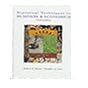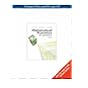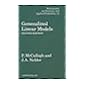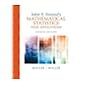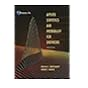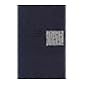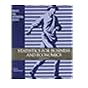519.5 MAS 1996 Statistical techniques in business and economics / 519.5 MAT 2008 Mathematical statistics with applications / 519.5 MCG 1989 Generalized linear models / 519.5 MIJ 2014 John E. Freund's mathematical statistics with applications / 519.5 MOA 2003 Applied statistics and probability for engineers / 519.5 MOI 1974 Introduction to the theory of statistics 519.5 NES 1995 Statistics for business & economics /

Includes bibliographical references and index.

TOC Introduction --
Probability --
Probability distributions and probability densities --
Mathematical expectation --
Special probability distributions --
Special probability densities --
Functions of random variables --
Sampling distributions --
Decision theory --
Point estimation --
Interval estimation --
Hypothesis testing --
Tests of hypothesis involving means, variances, and proportions --
Regression and correlation.

Summary:
John E. Freund's Mathematical Statistics with Applications, Eighth Edition, provides a calculus-based introduction to the theory and application of statistics, based on comprehensive coverage that Read more...

AS

Saifun Momota

There are no comments on this title.